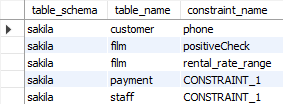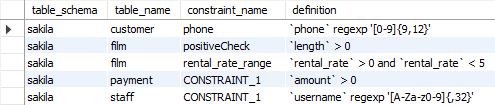## List table check constraints in MariaDB database

Article for:

The query below lists table check constraints.

### Notes

Before MariaDB 10.2.1 constraint expressions were accepted in the syntax but ignored. With MariaDB 10.2.1 comes check constraint support, but until MariaDB 10.3.10 and MariaDB 10.2.22 there was no possibility to obtain details.

If you are using one of these two versions check this query

### Query

``````select table_schema,
table_name,
constraint_name
from information_schema.table_constraints
where constraint_type = 'CHECK'
order by table_schema,
table_name;
``````

### Columns

• table_schema - database (schema) where the constraint is defined
• table_name - table name where the constraint is defined
• constraint_name - name of the constraint in the specified database (schema)

### Rows

• One row represents one check constraint
• Scope of rows: all check constraints in the specified database (schema)
• Ordered by database (schema), table name

### Sample results### Query

``````select constraint_schema as table_schema,
table_name,
constraint_name,
check_clause as definition
from information_schema.check_constraints
order by table_schema,
table_name;
``````

### Columns

• table_schema - database (schema) where the constraint is defined
• table_name - table where the constraint is defined
• constraint_name - name of the constraint
• definition - definition of the check constraint

### Rows

• One row represents one check constraint in table
• Scope of rows: all check constraints in the MariaDB database
• Ordered by databae (schema) name and table name

### Sample results